### Complex numbers and Newton fractals

Newton's method works just as well for complex numbers as for real numbers: sometimes finding a solution at blistering speed, and sometimes failing to work at all.

For instance, suppose we want to solve the equation $z^3 = 1$. There is just one real solution $z=1$, but over the complex numbers there are three solutions:

$z = 1, \; -\frac{1}{2} + \frac{\sqrt{3}}{2} i, \; - \frac{1}{2} - \frac{\sqrt{3}}{2} i.$

Starting from an initial point $z_1$, Newton's method works just as over the reals. We let $f(z) = z^3 - 1$, so $f'(z) = 3z^2$, and then calculate

$z_2 = z_1 - \frac{f(z_1)}{f'(z_1)} = z_1 - \frac{z^3 - 1}{3z^2}.$

From $z_2$, we calculate $z_3$, and then $z_4$, and so on.

We can ask: which choices of $z_1$ lead to which solutions? This leads to a very interesting picture. The picture below shows the complex plane, with real and imaginary axes labelled. For each point complex number $z$, we run Newton's method with $z_1 = z$, and see where the $z_n$ go. If they approach $-\frac{1}{2}+\frac{\sqrt{3}}{2} i$, we colour the point brown. If they approach $-\frac{1}{2} - \frac{\sqrt{3}}{2} i$, we colour the point blue. And if they approach $1$, we colour the point white.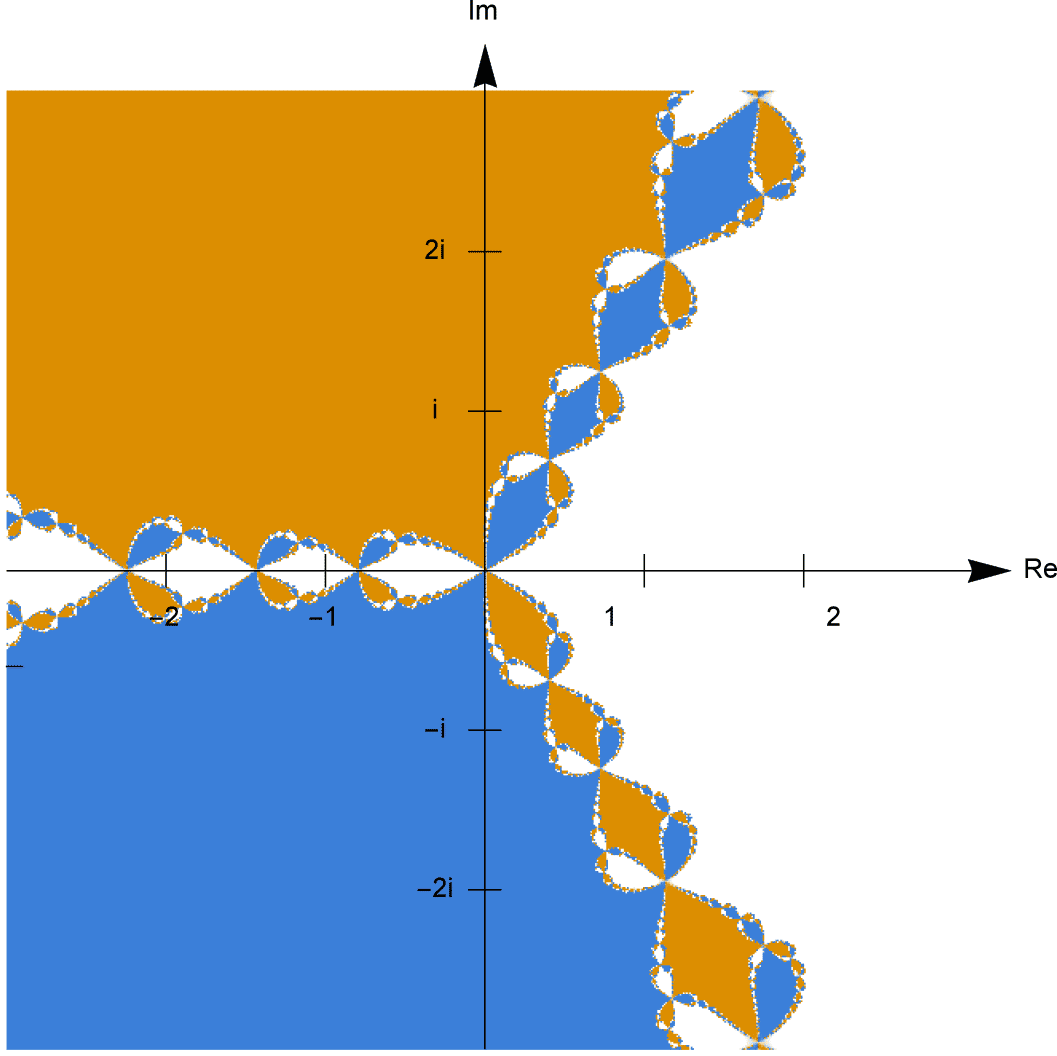We can see that, although there are large regions which converge to the three roots, there is an intricately complicated structure between these regions. We find complex numbers very close together, converging to different solutions, arranged in an intricate pattern.

In fact, if you zoom in on the details, you see even more detail. The more you zoom in, the more detail you see — and the details as you zoom in are similar to the details seen before you zoomed in. The pattern is self-similar, and is an object known as a fractal. For instance, if we zoom in on the square with corners $0, 1, i, 1+i$, we see an equally intricate picture.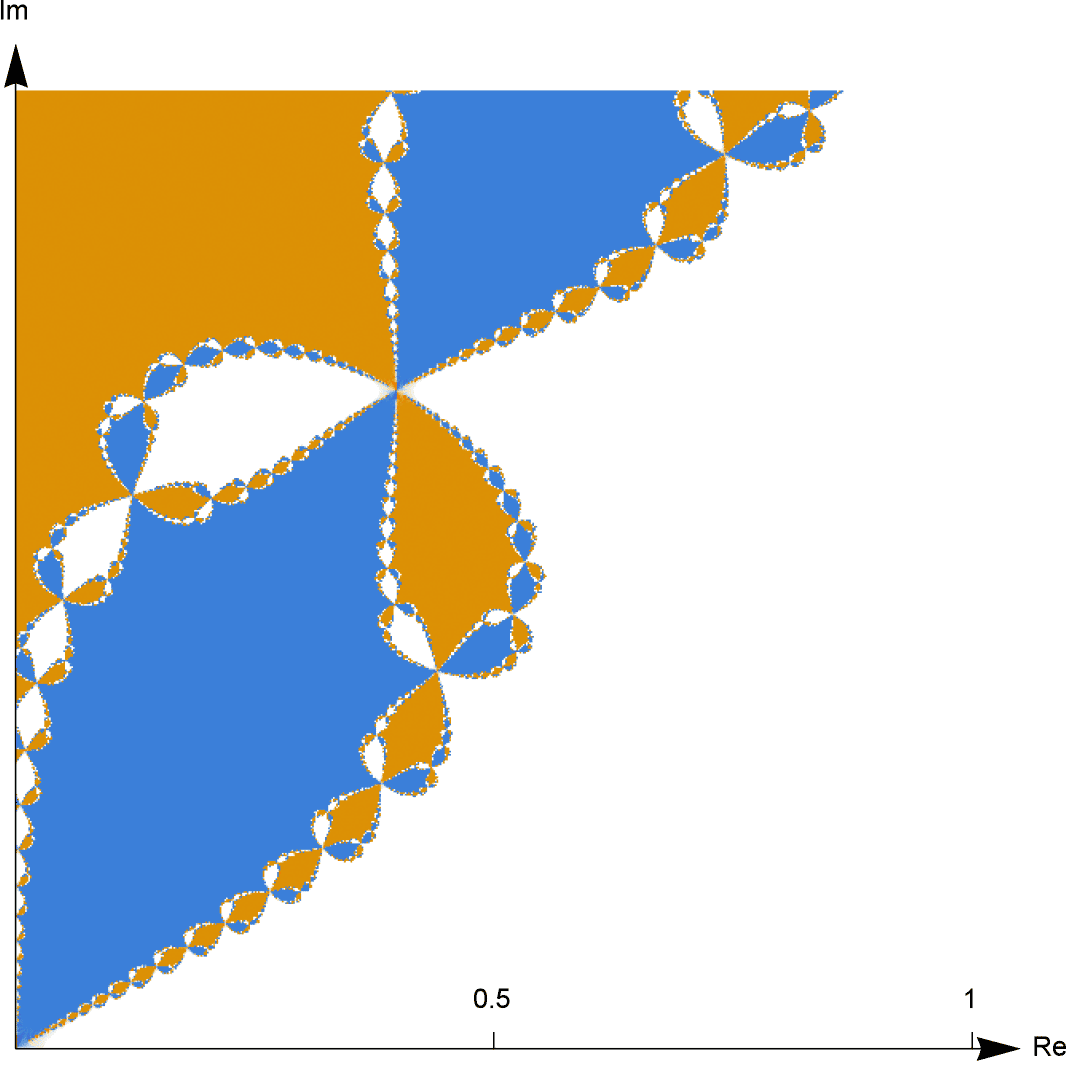The intricate arrangement of colours show just how sensitive Newton's method can be to initial conditions. The tiniest change in the initial estimate $x_1$ can move to a region of different colour, so that Newton's method leads to a completely different outcome.

Previously, we made a table showing outcomes when trying to solve the equation $2x - 3x^2 + x^3 = 0$. The solutions are $x=0,1,2$, but we found very sensitive dependence on initial conditions for $x_1$ between $1.4$ and $1.5$. In fact, the structure is much more intricate.

In the pictures below, points are coloured white, light brown, or orange, respectively as Newton's method approaches 0, 1 or 2. Each subsequent picture is obtained by repeatedly zooming in on the dotted square in the previous picture. The final picture shows the value of $1+\frac{1}{\sqrt{5}}$, which gives no solution but rather cycles between $2$ values.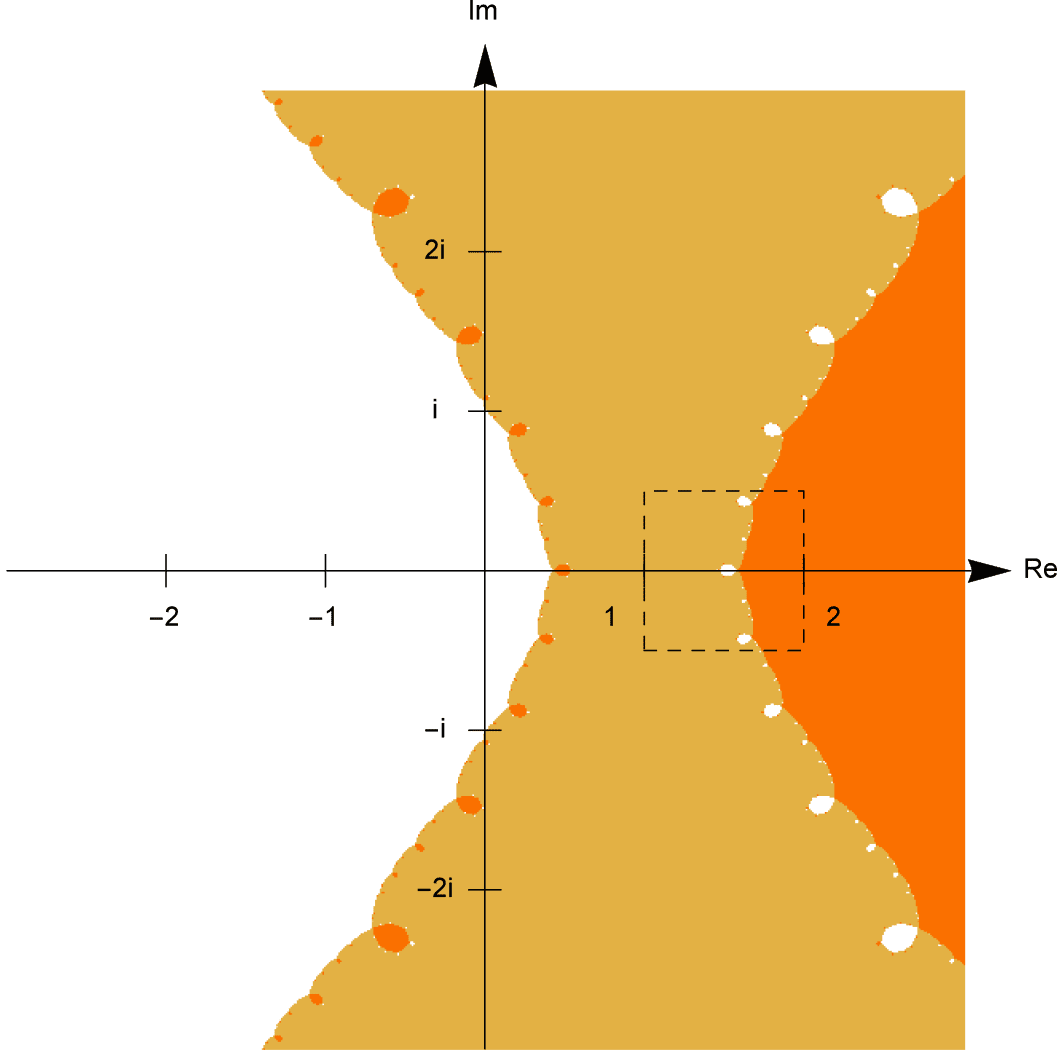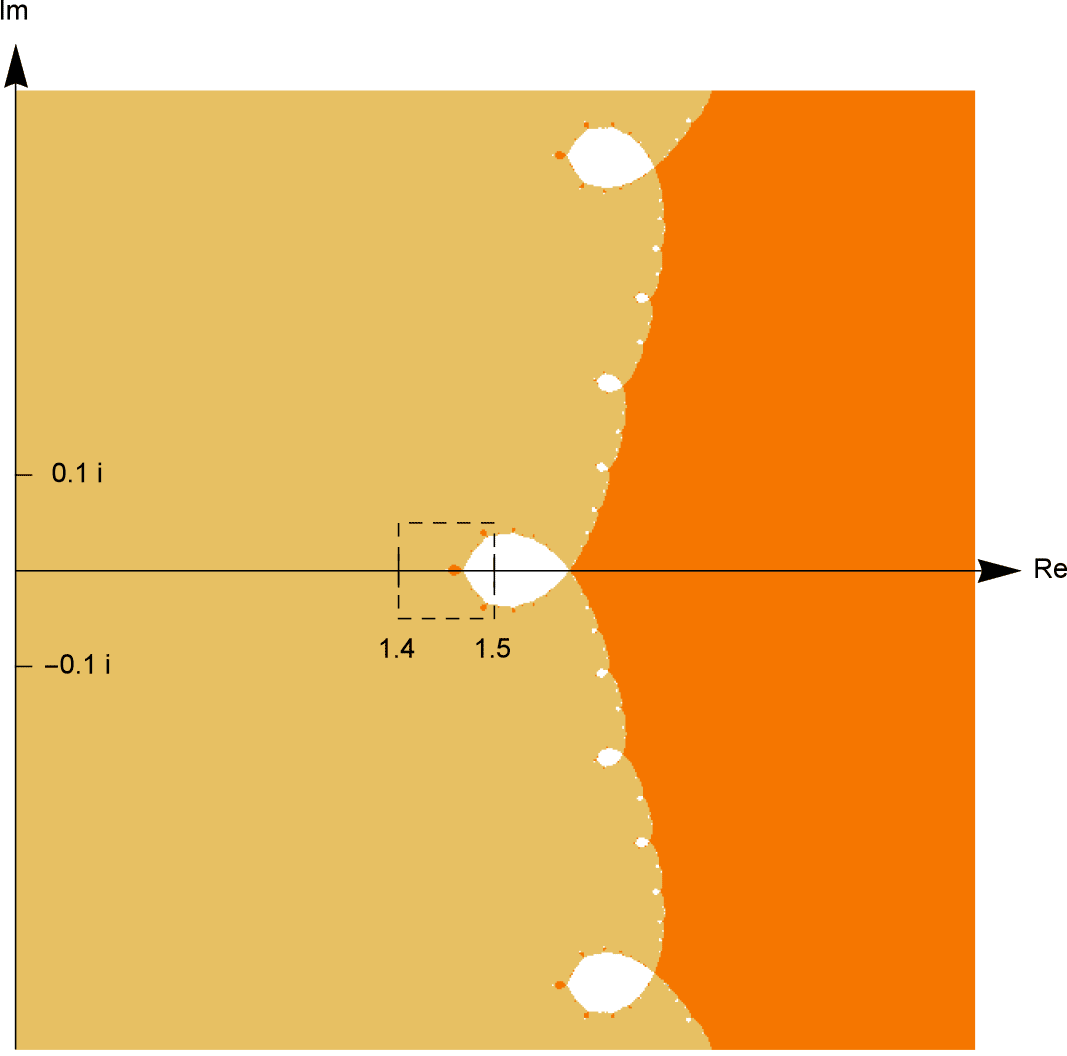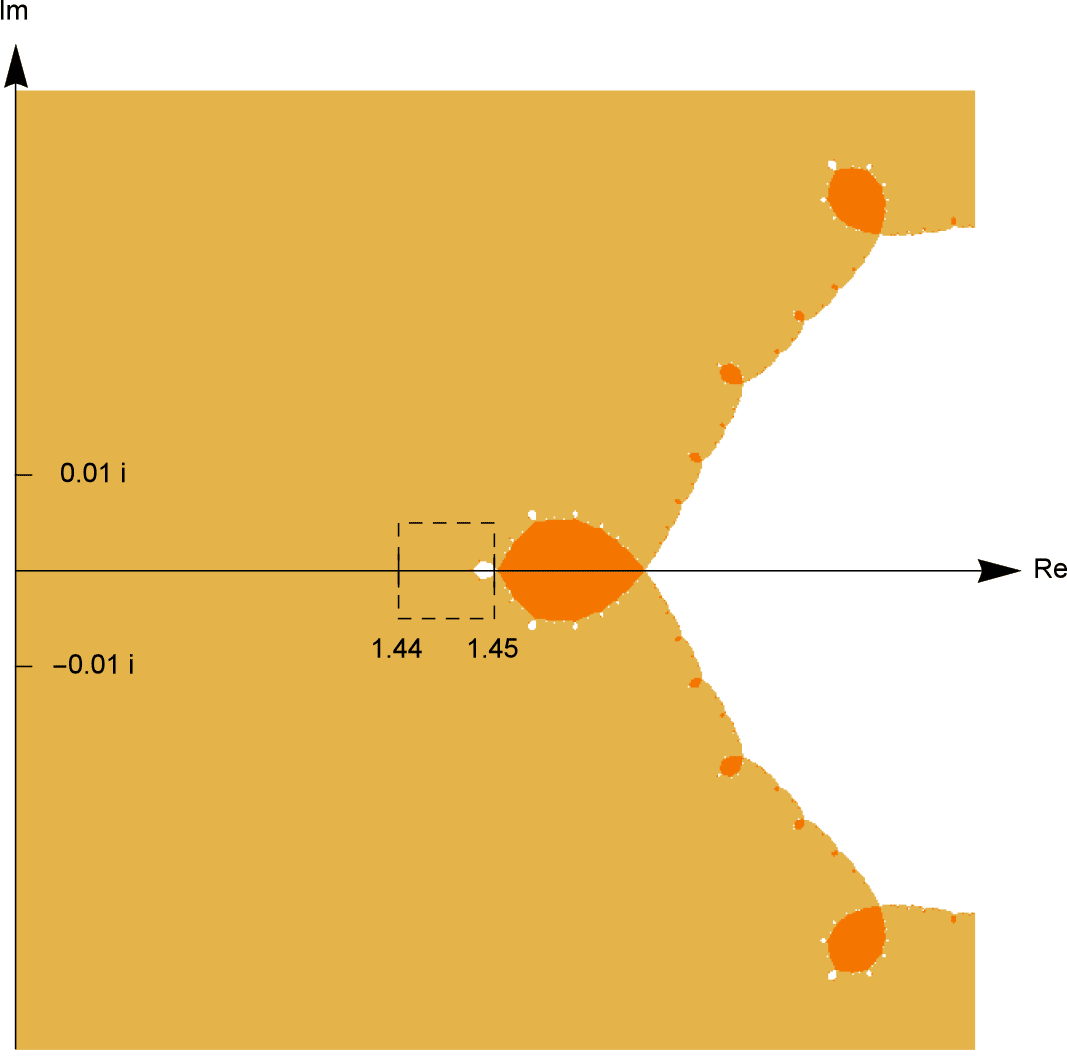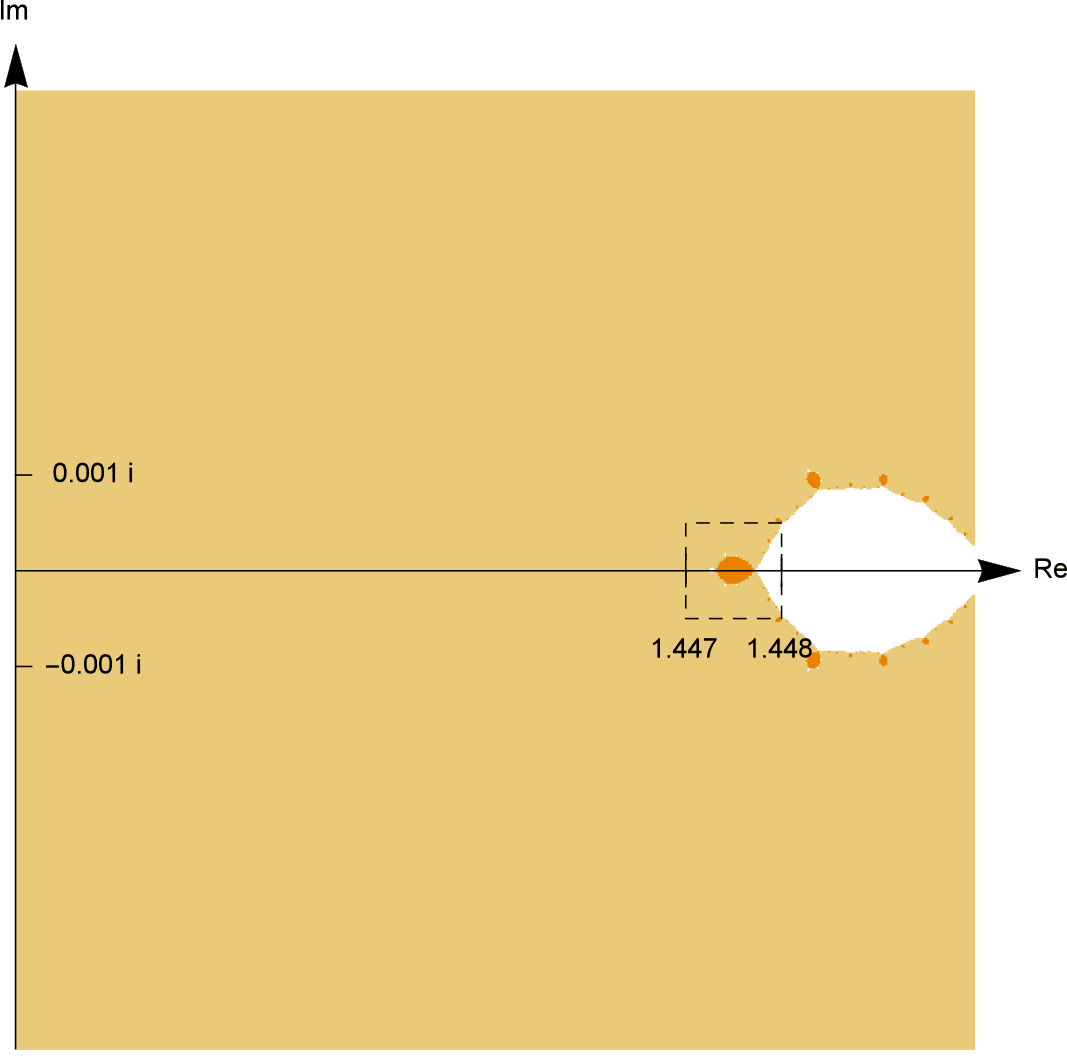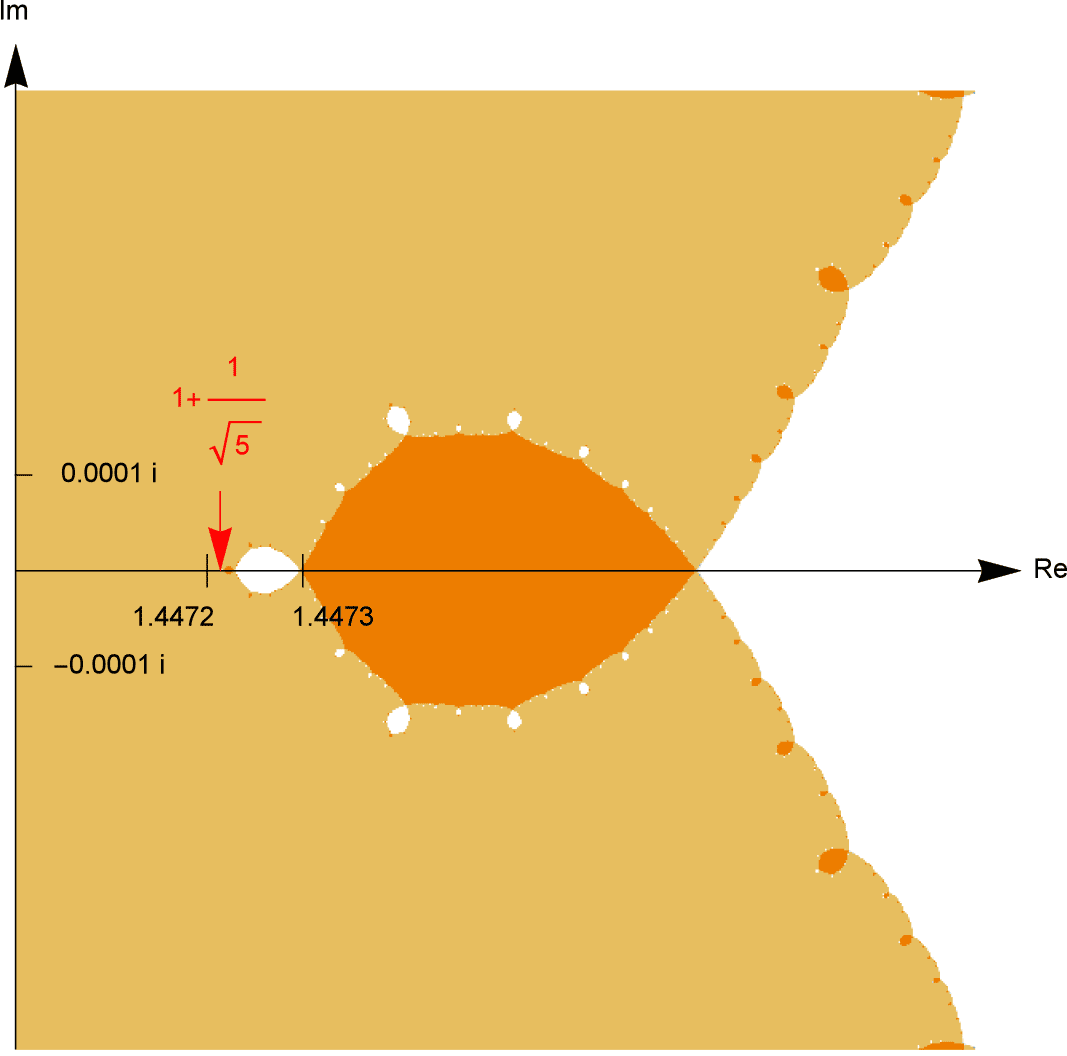Next page - Answers to exercises